# Order topology

(diff) ← Older revision | Latest revision (diff) | Newer revision → (diff)

The topology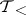on a linearly ordered set, with linear order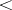, which has a base consisting of all possible intervals of.

Here "interval" is used in the sense of "open interval" , i.e. a set of the form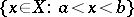where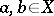(or possibly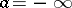and/or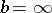). The order topology may be considered on partially ordered as well as linearly ordered sets; on a linearly ordered set it coincides with the interval topology which has the closed intervalsas a subbase for the closed sets, but in general it is different. On a complete linearly ordered set, the order topology is characterized by order convergence: that is, a net (see Generalized sequence)converges to a pointif and only if there are an increasing net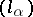and a decreasing net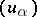, indexed by the same directed set, such thatfor alland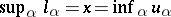.

How to Cite This Entry:
Order topology. Encyclopedia of Mathematics. URL: http://encyclopediaofmath.org/index.php?title=Order_topology&oldid=15011
This article was adapted from an original article by M.I. Voitsekhovskii (originator), which appeared in Encyclopedia of Mathematics - ISBN 1402006098. See original article